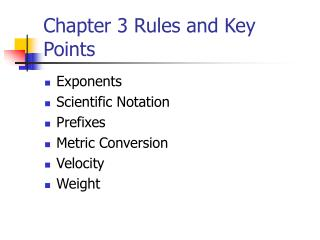Download PresentationChapter 3 Rules and Key Points

# Chapter 3 Rules and Key Points

Download Presentation## Chapter 3 Rules and Key Points

- - - - - - - - - - - - - - - - - - - - - - - - - - - E N D - - - - - - - - - - - - - - - - - - - - - - - - - - -
##### Presentation Transcript

1. Chapter 3 Rules and Key Points • Exponents • Scientific Notation • Prefixes • Metric Conversion • Velocity • Weight

2. Rule 3‑1: If we make the exponent more positive, the decimal point moves to the left. Rule 3‑2: If the exponent is made more negative, the decimal point moves to the right. 6700 = 670 X10 = 67.0 X 102 = 6.70 X 103 0.00275 = 0.0275 X10‑1 = 0.275 X 10-2 = 2.75 X 10‑3 Exponents

3. Key Point: Expressing numbers as numbers between 1 and 10 times the proper power of ten is called scientific notation. Expressing numbers as numbers times powers of ten that are multiples of 3 is called engineering notation. 47,000 = 4.70 X104 in scientific notation to three places. 47,000 = 47.0 X 103 inengineering notation to three places. Scientific Notation

4. Rule 3‑3: To change numbers with prefixes to regular numbers, replace the prefix with the power of ten that it represents and then change to a regular number. 30 mA = 30 X10‑3 A = 0.030 A 33 k = 33 X 103 = 33,000  Prefixes

5. Some conversions of linear measure between English and metric are: 1 in = 2.54 cm 1 cm = 0.3937 in 1 yd = 0.9144 m 1 m = 1.094 yd 1 mi = 1.609 km 1 km = 0.6214 mi 20 cm = 7.874 in12 in = 30.48 cm 100 m = 109.4 yd 5 mi = 8.045 km Metric Conversion

6. The units of velocity are meters/second or kilometers/hour in metric feet/second or miles/hour in English. 15 m/s = 49.22 ft/s Velocity

7. Common units of weight in metric are the milligram, gram, and kilogram. In the English system, we have the ounce, pound, and ton. 16 oz = 1 lb 1 kg = 35.27 oz 2000 lb = 1 ton 1 lb = 453.6 gm 100 lb = 45.36 kg 100 kg = 220.5 lb Weight